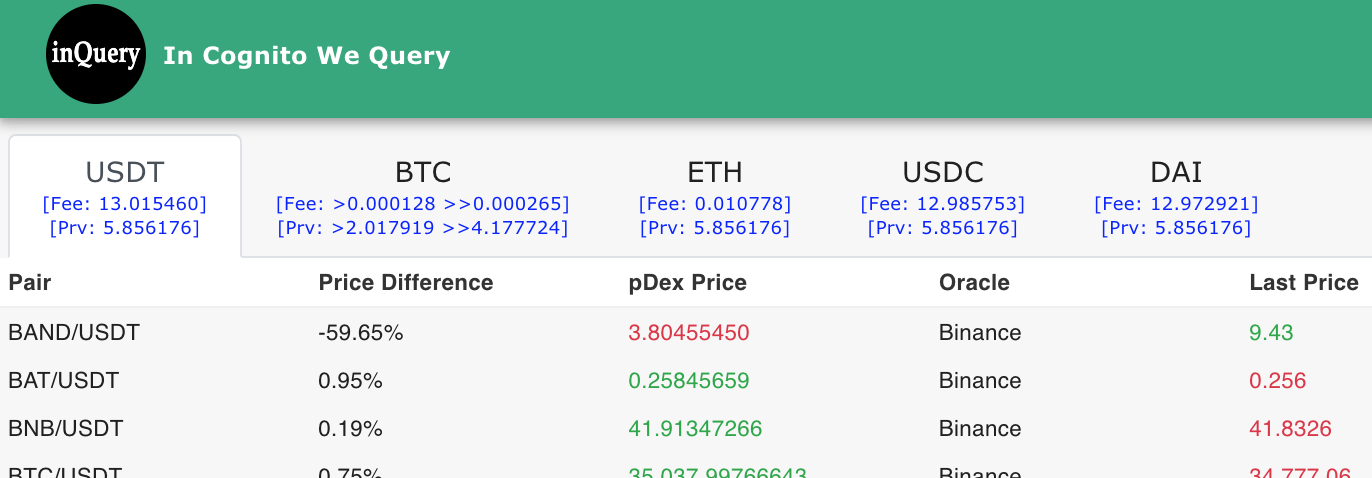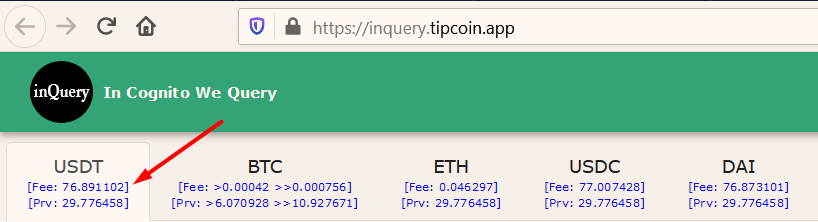# abduraman - InQuery - In Cognito We Query

Small update:

• Prv equivalents for the exit fees have been added.6 Likes

Sick update!! This ones gonna help a ton of users!

5 Likes

Hey @Support,

While examining data on inquery today, I’ve noticed that there are problems in https://api.incognito.org/ptoken/list. Because of that, pDex price is not accurate in inquery too.

Here are the problems:

• PricePrv is so wrong. For example, as of now, PRV/BTC is 15906 (which is correct acc. to what Incscan shows and my confirmation by calculation). However, ptokenlist shows 15817.

• I assume that you use the same USD calculation for all coins. In that case, for example, PriceUsd(BTC)/PriceUsd(ETH) should be equal to PricePrv(BTC)/PricePrv(ETH) but not so. In a similar manner, PriceUsd(BTC)/PricePrv(BTC) and PriceUsd(ETH)/PricePrv(ETH) should be equal but they are different too.

Only the correct value is CurrentPrvPool attribute but it is not enough for me to calculate the accurate prices among the coins. I need correct PricePrv values. Could you fix the script?

Thanks.

Note: I know that page is updated periodically. The problem is not about that. Price data are really outdated or have some calculation errors.

2 Likes

FYI @Support . There is another problem (which you may already know) besides the above. The address (ota/generate) which is used to calculate the exit fees of BTC frequently raises Internal Server Error (-9001) which is the reason for “can’t not get master address” error which is seen while the user tries to unshield BTC. The one for ETH and ERC-20 tokens is more stable.

A small grammatical error exists on the message too“can’t not” --> “can’t”

3 Likes

Hey @SupportDid you see my request?2 Likes

Hey @abduraman someone from the dev team will answer shortly.

3 Likes

hi @abduraman for current PRV price we calculate direct pool prv/pToken

I write example :
first get pdex state to get pool size via rpc :

``````{
"jsonrpc":"1.0",
"method":"getpdestate",
"params":[{
"BeaconHeight":<current value BeaconHeight>
}],
"id":1
}
``````

example result :

`//Pool Size PRV-pBTC "pdepool-962075-0000000000000000000000000000000000000000000000000000000000000004-b832e5d3b1f01a4f0623f7fe91d6673461e1f5d37d91fe78c5c2e6183ff39696": { "Token1IDStr": "0000000000000000000000000000000000000000000000000000000000000004", "Token1PoolValue": 2794646452014123, "Token2IDStr": "b832e5d3b1f01a4f0623f7fe91d6673461e1f5d37d91fe78c5c2e6183ff39696", "Token2PoolValue": 181299347091 },`

then cal for price pBTC in PRV Price :

``````    var decimal1 int64
var decimal2 int64

decimal1 = 9  // decimal PRV
decimal2 = 9 // decimal pBTC

var Token2PoolValue float64
var Token1PoolValue float64

Token1PoolValue = 2794646452014123 // PRV in pool size
Token2PoolValue = 181299347091  // pBTC in pool size

x2 := math.Ceil((Token2PoolValue *Token1PoolValue) / (Token2PoolValue + math.Pow10(int(decimal2))))
price := (Token1PoolValue - x2) / math.Pow10(int(decimal1))

fmt.Println(price)
``````

https://play.golang.org/p/LMXImgEkRhf

2 Likes

Why do you calculate the price in this way? What is the rationale behind it? @binh

Both incscan and my bots calculate the price just by dividing pool values:

``````price := Token1PoolValue * math.Pow10(-int(decimal1)) / (Token2PoolValue * math.Pow10(-int(decimal2)))
``````

So the results of incscan (any my products) and https://api.incognito.org/ptoken/list are different. To me, the correct one is inscan’s one. However, I want to know whether your code is buggy or has a rationale. Please could you clarify the issue? Or could you point out a link explaining your formula?

Thanks.

2 Likes

hey @abduraman, I just wanted to confirm that the formula @binh mentioned above is correct, so please update yours to that if possible, thanks.

2 Likes

What is the rationale behind it? @duc I cannot update the formula of Network Explorer since I’m not its developerI and @inccry calculate the price with the formula which I give. Could you want to join the discussion @inccry?

2 Likes

ah please have a look at the post as well as Automated Market Maker algorithm to understand the rationale behind it @abduraman

3 Likes

This explains why the deviation between your BTC/PRV index and Network Explorer’s BTC/PRV index is much higher than your ETH/PRV index and Network Explorer’s ETH/PRV index.

Imho @binh’s code has a small bug that does not affect the result. I’ll write it in the end. Let me try to formalize the difference between your calculation and Network Explorer’s calculation.

Let x, y, dx, dy be Token1PoolValue (PRV), Token2PoolValue (BTC), buy amount, and sell amount respectively.

I and Network Explorer calculate the price via `x/y` formula. As per Automated Market Maker algorithm document, you calculate the price via `dx/dy` formula. I confirmed this via the wallet app, too.

However, in your approach, imho there is a problem. For each coin, you assume that the user sells 1 unit of that coin, i.e. 1 BTC, 1 ETH, or 1 USDT etc. Here is the derivation.

``````x * y = (x - dx) * (y + dy)

(x * y) / (y + dy) = x - dx

dx = x  -  (x * y) / (y + dy)

Final formula: dx/dy = ( x  -  (x * y) / (y + dy) ) / dy
``````

Your code replaces `dy` with 1 unit of the corresponding coin in the formula as I wrote. So in fact, the prices in https://api.incognito.org/ptoken/list are the answers to “if the user sold 1 BTC, 1 ETH or 1 USDT, what would be the price?”. I think you will insist on this approach. No problem. I’ll update my code to show the prices compatible with Network Explorer.

Small bug: Since decimal1 and decimal2 have the same value, the results aren’t affected. However, `decimal1` in `price := (Token1PoolValue - x2) / math.Pow10(int(decimal1))` should be `decimal2` too. FYI.

3 Likes

I confirm, I won’t change Network Explorer calculations. It makes no sense on my side to show a price given a fixed bought/sold amount.

Maybe an extra feature for the Network Explorer : tell me how many coins you want to buy/sell and I’ll tell you the amount you’ll receive given the current pool state.

Thanks @abduraman for your great explanation!

4 Likesv1.0.4 releasedUpdate:

• As per the discussion above, the prices become compatible with Network Explorer. So they are much more accurate now.
5 Likes

I saw a post that this project shows unshielding fees without needing to check the wallet. Where is this? I’d find it extremely helpful as I hate the high fees!

Nevermind. I found it. This is very useful!4 Likes

Just discovered this feature heh, love this page

3 Likes

Maybe someone saw that you bought so many (pushing price up) and sold off a few, then you bought PRV back and the price had changed. You can look back at that time and see if that’s the case. Was this the case?

This tool was useful at day 0. Now this is even more helpful! @abduraman and @inccry, does it make sense to cross link between your two products to make it more useful for users to find the other tools? From a user perspective this seems like it would be helpful.

1 Like

Hey @RobynFitzooth,

I’m glad you enjoyed it.

As for your suggestion, I have a plan to make the main domain (tipcoin.app) a hub for both Tipcoin and Incognito Ecosystem but I need some time.

is that what impermenant loss is? when you are the buy then seller of two immediate transactions and 30% of value poofs into thin are like this?